• Hire UsUh Oh! It seems you’re using an Ad blocker!

We always struggled to serve you with the best online calculations, thus, there's a humble request to either disable the AD blocker or go with premium plans to use the AD-Free version for calculators.

Or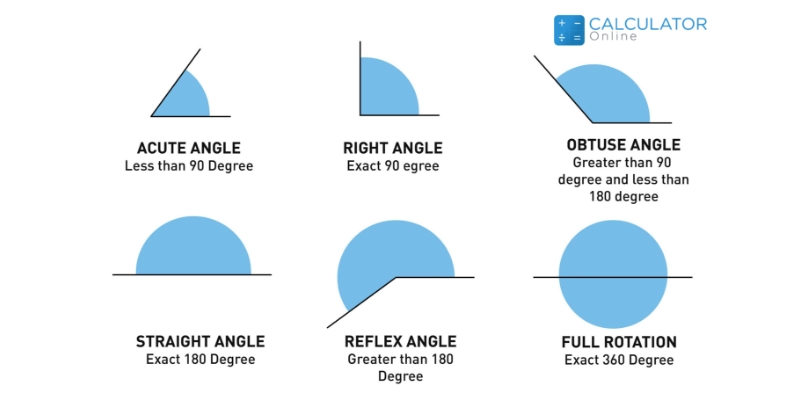# Types of Angles

## What is the angle?

In-plane geometry, an angle can be defined as:

“A figure formed by two rays or lines when combine with a common endpoint”

The word “angle” is carried out from the Latin word “angulus” which means “corner”. An angle has two components that include “sides” and “vertex”. Generally, the side can be described into terminal sides and initial sides (or vertical sides) as shown in the figure below.

### Parts of Angle:

Vertex:

A vertex is a corner point of an angle where two lines or sides are meet.

Arms:

The two straight sides of the angle where a common endpoint joined.

Initial Side:

A straight line where an angle is drawn known as the initial line.

Terminal Side:

The up-side where the angle measurement is done.

Also, you can use a double angle calculator to solve the trigonometric ratios like sin 2θ, cos 2θ, and tan 2θ.

### Types of Angles Based on Measurement:

Based on the measurement (magnitude), different types of angles in geometry are classified as follows:

#### Acute Angles:

“An angle that is less than 90 degrees or lies between 0 degrees and 90 degrees is termed an acute angle”.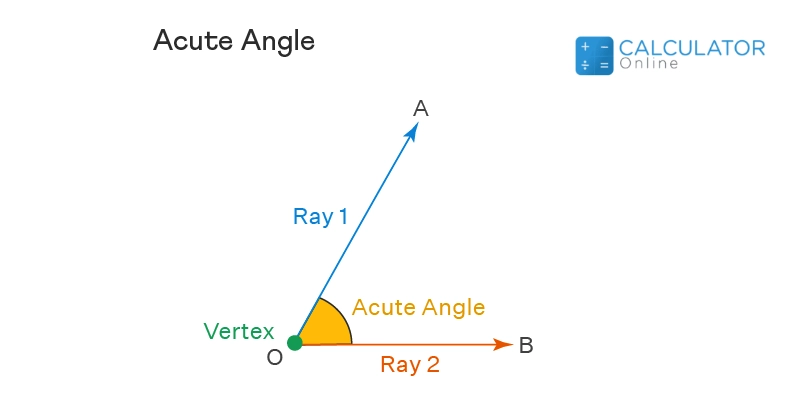#### Obtuse angle:

Now you must think about what is an obtuse angle. Read below to know about the obtuse angle definition that is one of the different types of angles in geometry and is given as:
“An angle that is greater than 90 degrees but less than 180 degrees or lies between 90 degrees and 180 degrees is known as the obtuse angle”.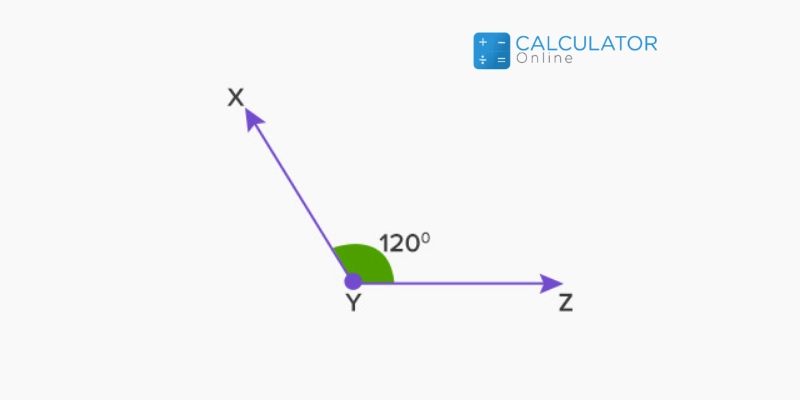#### Right angle:

“An angle that is equal to 90 degrees is called a right angle. Since it is one of the angle types geometry in math that represent 90 degrees”.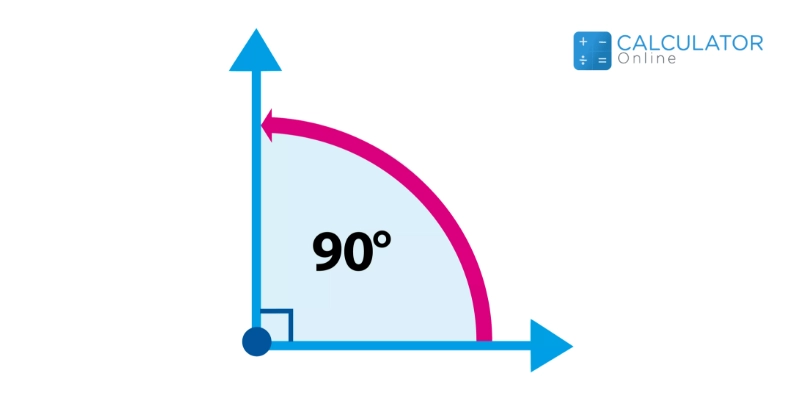#### Straight angle:

Whether you want to know what is a straight angle. Let’s discuss the definition of straight angle that is given below:
“An angle that measures equal to 180 degrees is termed a straight angle.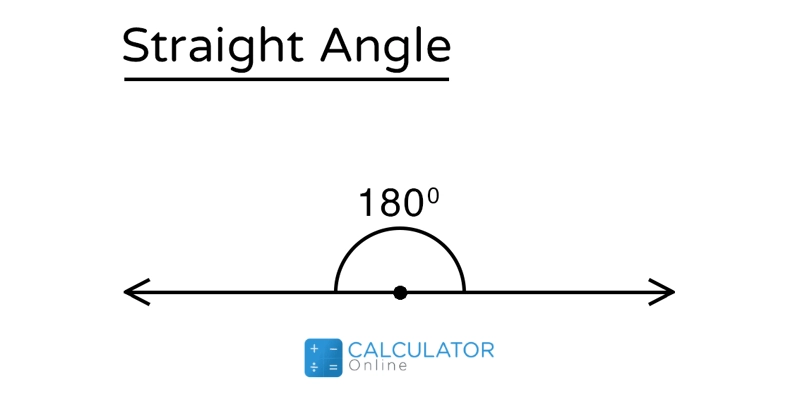#### Reflex angle:

“The angle that is greater than 180 degrees and less than 360 degrees is called a reflex angle”.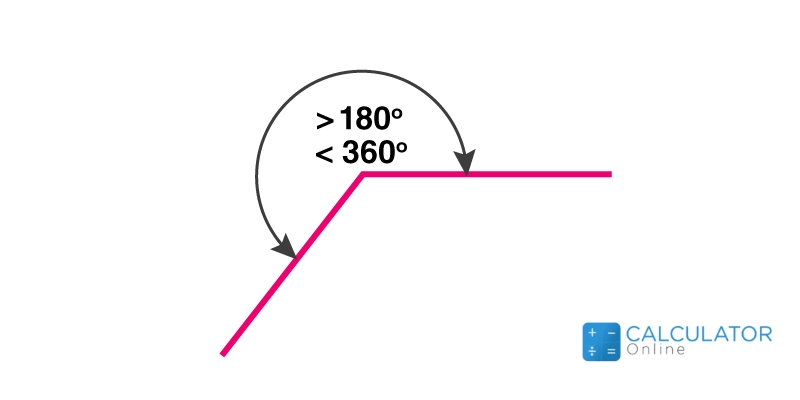#### Full rotation angle:

“An angle whose measurement is equal to 360 degrees is known as full rotation or full angle”. This angle is formed when one of the arms takes a complete rotation.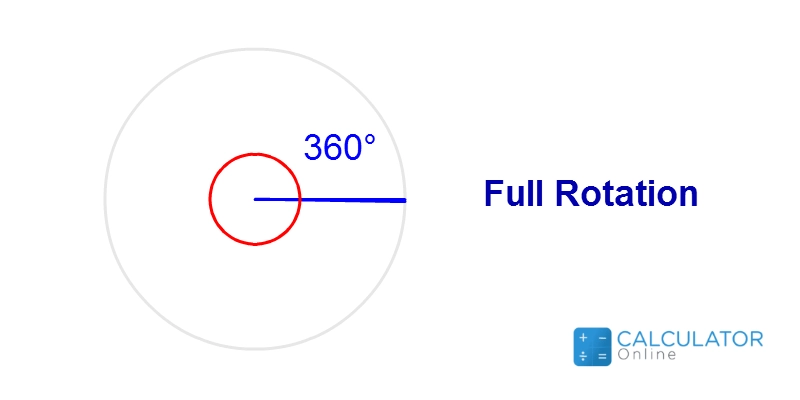### Types of angles Based on Rotation:

Based on the direction of the rotation, different angles in geometry can be categorised as follow:

#### Positive Angles:

Since it is one of the angle types geometry where angles are measured (rotated) in a counterclockwise direction from the base. Commonly, these angles are used to represent geometry angles names in math. If an angle from the origin is drawn in the (+x, +y) plane, it makes a positive angle.

#### Negative Angles:

It is one of all types of angles in math in which angles are measured in a clockwise direction from the base. These angles are commonly used to describe geometry angles names. If an angle is drawn towards the (x, -y) plane from the origin, a negative angle is formed.
Use an online half angle calculator to find the trigonometric values for angle θ/2 with respect to θ with the expression of different trigonometric functions.

### Pair of Angles:

When two angles are paired, different angles in math are formed. These are:

#### Complementary angles:

An angle is said to be complementary when the sum of two angles is equal to 90°.

Example: Two angles such as  30° and 60°make up 90°

#### Supplementary Angles:

When the sum of two angles is equal to 180°, they are said to be supplementary angles.

Example: when two angles like  110° and 70°added, they make up 180°.

#### Alternate Interior Angles:

When a line or transversal intersects two parallel lines, equal angles are formed at the opposite (inner) sides which are called alternate interior angles. Since it is one of the different angles in geometry.

#### Alternate Exterior Angles:

When a line or a transversal intersects two parallel lines, they form equal angles at the external side of the line which is known as alternate exterior angles.

#### Corresponding Angles:

The angles that occur at the same position or on the same side of the line or transversal when intersecting two parallel lines are called corresponding angles and these angles are congruent.

#### Vertical Angles:

When two lines intersect each other, the equal angles are opposite to each other and are called vertical angles. So it belongs to different kinds of angles in geometry.